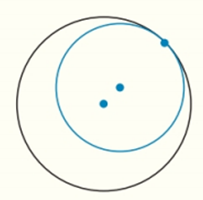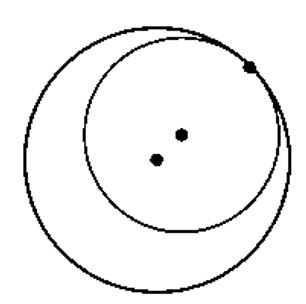Chapter 6.CT, Problem 10CTElementary Geometry For College St...

7th Edition
Alexander + 2 others
ISBN: 9781337614085

Solutions

Chapter
SectionElementary Geometry For College St...

7th Edition
Alexander + 2 others
ISBN: 9781337614085
Textbook Problem

For the circles described and shown, how many common tangents do they possess?a) Internally tangent circlesb) Circles that intersect in two pointsTo determine

a)

To find:

The number of common tangents in internally tangent circles.Explanation

Given:

The internally tangent circles.

Definition used:

A line, line segment, or ray that is tangent to each of two circles is a common tangent for the given circles. If the common tangent does not intersect the line segment joining the centers, it is a common external tangent.

Calculation:

Consider,

To determine

b)

The number of common tangents in circles that intersect in two points,Still sussing out bartleby?

Check out a sample textbook solution.

See a sample solution

The Solution to Your Study Problems

Bartleby provides explanations to thousands of textbook problems written by our experts, many with advanced degrees!

Get Started

Evaluate the expression sin Exercises 116. (23)2

Finite Mathematics and Applied Calculus (MindTap Course List)

Finding Derivatives In Exercises 51-62, find f(x). f(x)=x24x3x2

Calculus: An Applied Approach (MindTap Course List)

Let g be the function defined by g(x)={12x+1ifx2x2ifx2 Find g(2), g(0), g(2), and g(4).

Applied Calculus for the Managerial, Life, and Social Sciences: A Brief Approach

In Exercises 1-8, construct a truth table for each compound proposition. ~(~p)

Finite Mathematics for the Managerial, Life, and Social Sciences